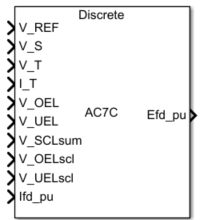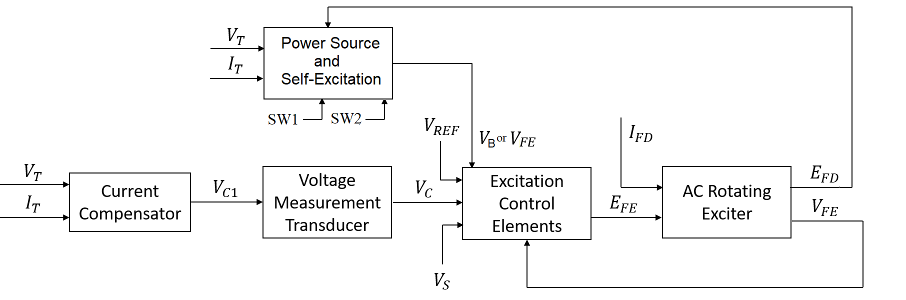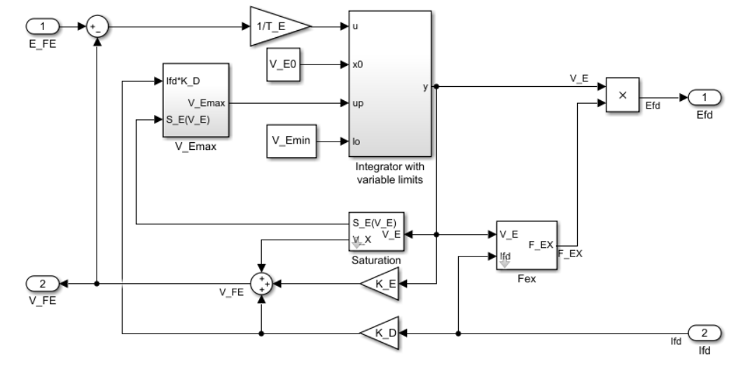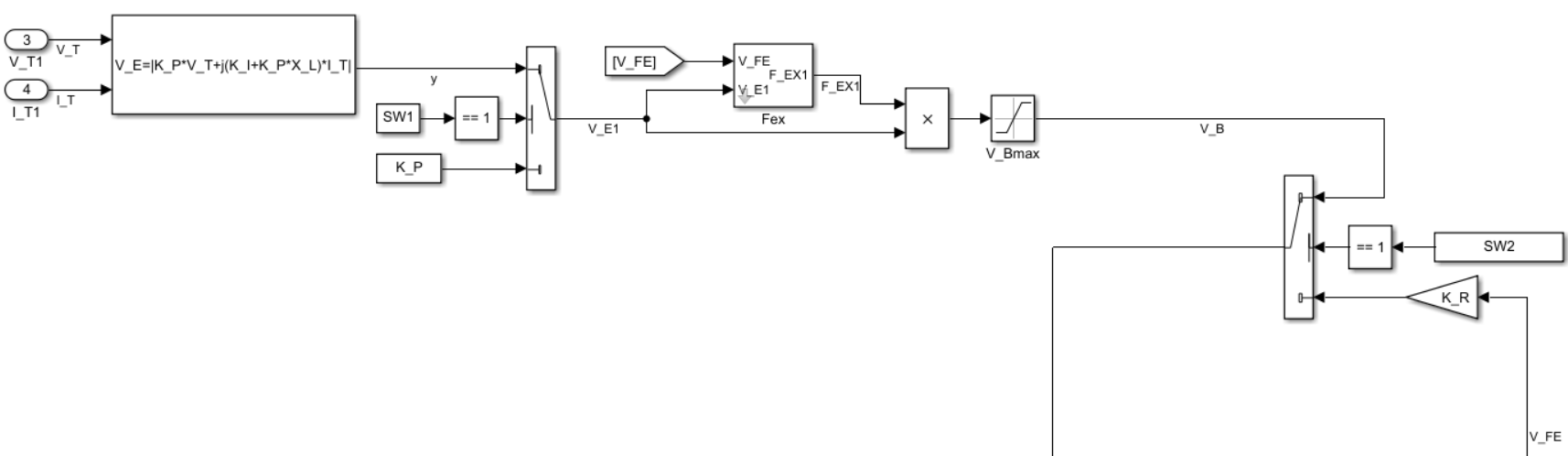# SM AC7C

Discrete-time or continuous-time synchronous machine AC7C excitation system including an automatic voltage regulator and an exciter

• Library:
• Simscape / Electrical / Control / SM Control

•## Description

The SM AC7C block implements a synchronous machine type AC7C excitation system model in conformance with IEEE 421.5-2016.

Use this block to model the control and regulation of the field voltage of a synchronous machine that operates as a generator using an AC rotating exciter.

You can switch between continuous and discrete implementations of the block by using the Sample time (-1 for inherited) parameter. To configure the integrator for continuous time, set the Sample time (-1 for inherited) property to `0`. To configure the integrator for discrete time, set the Sample time (-1 for inherited) property to a positive, nonzero value, or to `-1` to inherit the sample time from an upstream block.

The SM AC7C block is made up of five major components:

• The Current Compensator modifies the measured terminal voltage as a function of the terminal current.

• The Voltage Measurement Transducer simulates the dynamics of a terminal voltage transducer using a low-pass filter.

• The Excitation Control Elements component compares the voltage transducer output with a terminal voltage reference to produce a voltage error. This voltage error is then passed through a voltage regulator to produce the exciter field voltage.

• The AC Rotating Exciter models the AC rotating exciter, which produces a field voltage that is applied to the controlled synchronous machine. The block also feeds the exciter field current (which is given the standard symbol VFE) back to the excitation system.

• The Power Source and Self-excitation models the power source for the controlled rectifier when it is independent from the terminal voltage. It also allows the representation of self-excitation for the exciter.

This diagram shows the overall structure of the AC7C excitation system model:In the diagram:

• VT and IT are the measured terminal voltage and current of the synchronous machine.

• VC1 is the current-compensated terminal voltage.

• VC is the filtered, current-compensated terminal voltage.

• VREF is the reference terminal voltage.

• VS is the power system stabilizer voltage.

• SW1 and SW2 are the user-selected power source switches for the controlled rectifier.

• VB is the exciter field voltage.

• EFE and VFE are the exciter field voltage and current, respectively.

• EFD and IFD are the field voltage and current, respectively.

The following sections describe each of the major parts of the block in detail.

### Current Compensator and Voltage Measurement Transducer

The current compensator is modeled as:

`${V}_{C1}={V}_{T}+{I}_{T}\sqrt{{R}_{C}^{2}+{X}_{C}^{2}},$`

where:

• RC is the load compensation resistance.

• XC is the load compensation reactance.

The voltage measurement transducer is implemented as a Low-Pass Filter block with time constant TR. Refer to the documentation for this block for the discrete and continuous implementations.

### Excitation Control Elements

This diagram illustrates the overall structure of the excitation control elements:In the diagram:

• The Summation Point Logic subsystem models the summation point input location for the overexcitation limiter (OEL), underexcitation limiter (UEL), stator current limiter (SCL), and the power switch selector (V_S) voltages. For more information about using limiters with this block, see Field Current Limiters.

• There are three Take-over Logic subsystems. They model the take-over point input locations for the OEL, UEL, SCL and PSS voltages. For more information about using limiters with this block, see Field Current Limiters.

• The PID_R subsystem models a PID controller that functions as a control structure for the automatic voltage regulator. The minimum and maximum anti-windup saturation limits for the block are VRmin and VRmax, respectively.

• The PI_R subsystem models a PI controller that functions as a control structure for the field current regulator. The minimum and maximum anti-windup saturation limits for the block are VAmin and VAmax, respectively.

• In case of self-excitation, with a nonzero KR and the Power source selector SW2 parameter set to `Position B: do not use variable V_B`, the voltage regulator power is derived from the exciter output voltage. The voltage regulator command signal is multiplied by the exciter output voltage, EFD, times KR. For more information about the user-selected logical switches for the power source of the controlled rectifier, see Power Source and Self-Excitation.

• KF1 and KF2 model a high-bandwidth regulator inner loop that regulates the generator field or exciting current.

• The Filtered Derivative block models the rate feedback path for the stabilization of the excitation system if the automatic voltage regulator does not have a derivative term. Here, KF3 and TF are the gain and time constants of this system, respectively. Refer to the documentation for the Filtered Derivative block for the discrete and continuous implementations.

• -K_L*VFE and inf are the minimum and maximum saturation limits for the output exciter field voltage EFE.

### Field Current Limiters

You can use various field current limiters to modify the output of the voltage regulator under unsafe operating conditions:

• Use an overexcitation limiter to prevent overheating of the field winding due to excessive field current demand.

• Use an underexcitation limiter to boost field excitation when it is too low, which risks desynchronization.

• Use a stator current limiter to prevent overheating of the stator windings due to excessive current.

Attach the output of any of these limiters at one of these points:

• The summation point as part of the automatic voltage regulator (AVR) feedback loop

• The take-over point to override the usual behavior of the AVR

If you are using the stator current limiter at the summation point, use the single input VSCLsum. If you are using the stator current limiter at the take-over point, use both the overexcitation input, VOELscl, and the underexcitation input, VUELscl.

### AC Rotating Exciter

This diagram illustrates the overall structure of the AC rotating exciter:In the diagram:

• The exciter field current VFE is modeled as the summation of three signals:

• The nonlinear function Vx models the saturation of the exciter output voltage.

• The proportional term KE models the linear relationship between exciter output voltage and the exciter field current.

• The demagnetizing effect of the load current on the exciter output voltage is modeled using the demagnetization constant KD in the feedback loop.

• The Integrator with variable limits subsystem integrates the difference between EFE and VFE to generate the exciter alternator output voltage VE. TE is the time constant for this process.

• The nonlinear function FEX models the exciter output voltage drop from the rectifier regulation. This function depends on the constant KC, which itself is a function of commutating reactance.

• The parameters VEmin and VFEmax model the lower and upper limits of the rotating exciter.

### Power Source and Self-Excitation

It is possible to use different power source representations for the controlled rectifier by selecting the relevant option in the Power source selector SW1 parameter. The power source for the controlled rectifier can be either derived from the terminal voltage (```Position A: power source derived from terminal voltage```) or it can be independent of the terminal voltage (```Position B: power source independent from the terminal conditions```). Note that this switch is dependent on the state of the second logical switch.

The second logical switch, Power source selector SW2, determines whether the exciter employs self-excitation or not. The exciter is self-excited if the Power source selector SW2 parameter is set to `Position B: do not use variable V_B`, as the model parameters associated with the variable VB do not impact the simulation.## Ports

### Input

expand all

Voltage regulator reference set point, in per-unit representation, specified as a scalar.

Data Types: `single` | `double`

Input from the power system stabilizer, in per-unit representation, specified as a scalar.

Data Types: `single` | `double`

Terminal voltage magnitude in per-unit representation, specified as a scalar.

Data Types: `single` | `double`

Terminal current magnitude in per-unit representation, specified as a scalar.

Data Types: `single` | `double`

Input from the overexcitation limiter, in per-unit representation, specified as a scalar.

#### Dependencies

• To ignore the input from the overexcitation limiter, set Alternate OEL input locations (V_OEL) to `Unused`.

• To use the input from the overexcitation limiter at the summation point, set Alternate OEL input locations (V_OEL) to ```Summation point```.

• To use the input from the overexcitation limiter at the take-over point, set Alternate OEL input locations (V_OEL) to `Take-over`.

Data Types: `single` | `double`

Input from the underexcitation limiter, in per-unit representation, specified as a scalar.

#### Dependencies

• To ignore the input from the underexcitation limiter, set Alternate UEL input locations (V_UEL) to `Unused`.

• To use the input from the underexcitation limiter at the summation point, set Alternate UEL input locations (V_UEL) to ```Summation point```.

• To use the input from the underexcitation limiter at the take-over point, set Alternate UEL input locations (V_UEL) to `Take-over`.

Data Types: `single` | `double`

Input from the stator current limiter when using the summation point, in per-unit representation, specified as a scalar.

#### Dependencies

• To ignore the input from the stator current limiter, set Alternate SCL input locations (V_SCL) to `Unused`.

• To use the input from the stator current limiter at the summation point, set Alternate SCL input locations (V_SCL) to ```Summation point```.

Data Types: `single` | `double`

Input from the stator current limiter to prevent field overexcitation when using the take-over point, in per-unit representation, specified as a scalar.

#### Dependencies

• To ignore the input from the stator current limiter, set Alternate SCL input locations (V_SCL) to `Unused`.

• To use the input from the stator current limiter at the take-over point, set Alternate SCL input locations (V_SCL) to `Take-over`.

Data Types: `single` | `double`

Input from the stator current limiter to prevent field underexcitation when using the take-over point, in per-unit representation, specified as a scalar.

#### Dependencies

• To ignore the input from the stator current limiter, set Alternate SCL input locations (V_SCL) to `Unused`.

• To use the input from the stator current limiter at the take-over point, set Alternate SCL input locations (V_SCL) to `Take-over`.

Data Types: `single` | `double`

Measured per-unit field current of the synchronous machine, specified as a scalar.

Data Types: `single` | `double`

### Output

expand all

Per-unit field voltage to apply to the field circuit of the synchronous machine, returned as a scalar.

Data Types: `single` | `double`

## Parameters

expand all

### General

Initial per-unit voltage to apply to the field circuit of the synchronous machine.

Time between consecutive block executions. During execution, the block produces outputs and, if appropriate, updates its internal state. For more information, see What Is Sample Time? and Specify Sample Time.

For inherited discrete-time operation, specify `-1`. For discrete-time operation, specify a positive integer. For continuous-time operation, specify `0`.

If this block is in a masked subsystem, or other variant subsystem that allows you to switch between continuous operation and discrete operation, promote the sample time parameter. Promoting the sample time parameter ensures correct switching between the continuous and discrete implementations of the block. For more information, see Promote Block Parameters on a Mask.

### Pre-Control

Resistance used in the current compensation system. Set this parameter and Reactance component of load compensation, X_C (pu) to `0` to disable current compensation.

Reactance used in the current compensation system. Set this parameter and Resistive component of load compensation, R_C (pu) to `0` to disable current compensation.

Equivalent time constant for the voltage transducer filtering.

### Control

Per-unit proportional gain of the voltage regulator.

Per-unit integral gain of the voltage regulator.

Derivative gain of the voltage regulator.

Equivalent lag time constant for the derivative channel of the PID controller.

Maximum per-unit output voltage of the regulator.

Minimum per-unit output voltage of the regulator.

Per-unit proportional gain associated with the field current regulator.

Per-unit integral gain associated with the field current regulator.

Maximum per-unit field current regulator output.

Minimum per-unit field current regulator output.

Per-unit field voltage feedback gain associated with the generator.

Per-unit field current feedback gain associated with the exciter.

Per-unit rate feedback block gain.

Rate feedback block time constant for the stabilization of the excitation system.

Location of the power system stabilizer input.

Location of the overexcitation limiter input:

• If you select ```Summation point at voltage error```, V_OEL is an input of the Summation Point Logic subsystem.

• If you select ```Take-over at voltage error```, V_OEL is an input of the Take-over Logic subsystem.

• If you select ```Take-over at voltage regulator output```, V_OEL is an input of the Take-over Logic 1 subsystem.

• If you select ```Take-over at output of inner loop regulator```, V_OEL is an input of the Take-over Logic 2 subsystem.

Location of the underexcitation limiter input:

• If you select ```Summation point at voltage error```, V_UEL is an input of the Summation Point Logic subsystem.

• If you select ```Take-over at voltage error```, V_UEL is an input of the Take-over Logic subsystem.

• If you select ```Take-over at voltage regulator output```, V_UEL is an input of the Take-over Logic 1 subsystem.

Loction of the stator current limiter input:

• If you select `Summation point`, use the V_SCLsum input port.

• If you select any of the `Take-over` options, use the V_OELscl and V_UELscl input ports.

### Exciter

Proportional constant for the exciter field.

Time constant for the exciter field.

Demagnetization factor related to the exciter alternator reactances.

Exciter output voltage for the first saturation factor.

Saturation factor for the first exciter.

Exciter output voltage for the second saturation factor.

Saturation factor for the second exciter.

Maximum per-unit field current limit reference.

Minimum per-unit exciter voltage output.

Per-unit potential circuit gain coefficient.

#### Dependencies

This parameter is visible only when you set the Power source selector SW1 parameter to ```Position A: power source derived from terminal voltage``` and the Power source selector SW2 parameter to ```Position A: use variable V_B```.

Potential circuit phase angle, in degrees.

#### Dependencies

To enable this parameter, set Power source selector SW1 to ```Position A: power source derived from terminal voltage``` and Power source selector SW2 to ```Position A: use variable V_B```.

Per-unit potential circuit current gain coefficient.

#### Dependencies

To enable this parameter, set Power source selector SW1 to ```Position A: power source derived from terminal voltage``` and Power source selector SW2 to ```Position A: use variable V_B```.

Per-unit reactance associated with the potential source.

#### Dependencies

To enable this parameter, set Power source selector SW1 to ```Position A: power source derived from terminal voltage``` and Power source selector SW2 to ```Position A: use variable V_B```.

Per-unit gain related to the regulator and alternator field power supply.

#### Dependencies

To enable this parameter, set Power source selector SW2 to ```Position B: do not use variable V_B```.

Per-unit gain related to the negative exciter field current capability.

Position of the power source selector SW1.

#### Dependencies

To enable this parameter, set Power source selector SW2 to `Position A: use variable V_B`.

Position of the power source selector SW2.

 IEEE Recommended Practice for Excitation System Models for Power System Stability Studies. IEEE Std 421.5-2016. Piscataway, NJ: IEEE-SA, 2016.